# Getting started with wrGraph

## Introduction

This package contains a collection of various plotting tools, mostly as an extension of packages wrMisc and wrProteo.

This package is available from CRAN, you might also have to install wrMisc, too. If not yet installed, the lastest versions of this package can be installed like this :

``install.packages("wrGraph")``

During this vignette we’ll also use the packages FactoMineR and factoextra, let’s test if they are installed and install if not yet present.

``````if(!requireNamespace("FactoMineR", quietly=TRUE)) install.packages("FactoMineR")
if(!requireNamespace("factoextra", quietly=TRUE)) install.packages("factoextra")``````

You cat start the vignette for this package by typing :

``````# access vignette :
browseVignettes("wrGraph")    #  ... and the select the html output``````

To get started, we need to load the packages wrMisc and wrGraph (this package).

``````library("wrMisc")
library("wrGraph")
# This is version no:
packageVersion("wrGraph")
#>  '1.3.1'``````

## Prepare Layout for Accomodating Multiple Smaller Plots

The function partitionPlot() prepares a matrix to serve as grid for segmenting the current device (ie the available plotting region). It aims to optimize the layout based on a given number of plots to accommodate. The user may choose if the layout should rather be adopted to landscape (default) or portrait geometry.

This might be useful in particular when during an analysis-pipeline it’s not known/clear in advance how many plots might be needed in a single figure space.

``````## as the last column of the Iris-data is not numeric we choose -1
(part <- partitionPlot(ncol(iris)-1))
#>      [,1] [,2]
#> [1,]    1    2
#> [2,]    3    4
layout(part)
for(i in colnames(iris)[-5]) hist(iris[,i], main=i)``````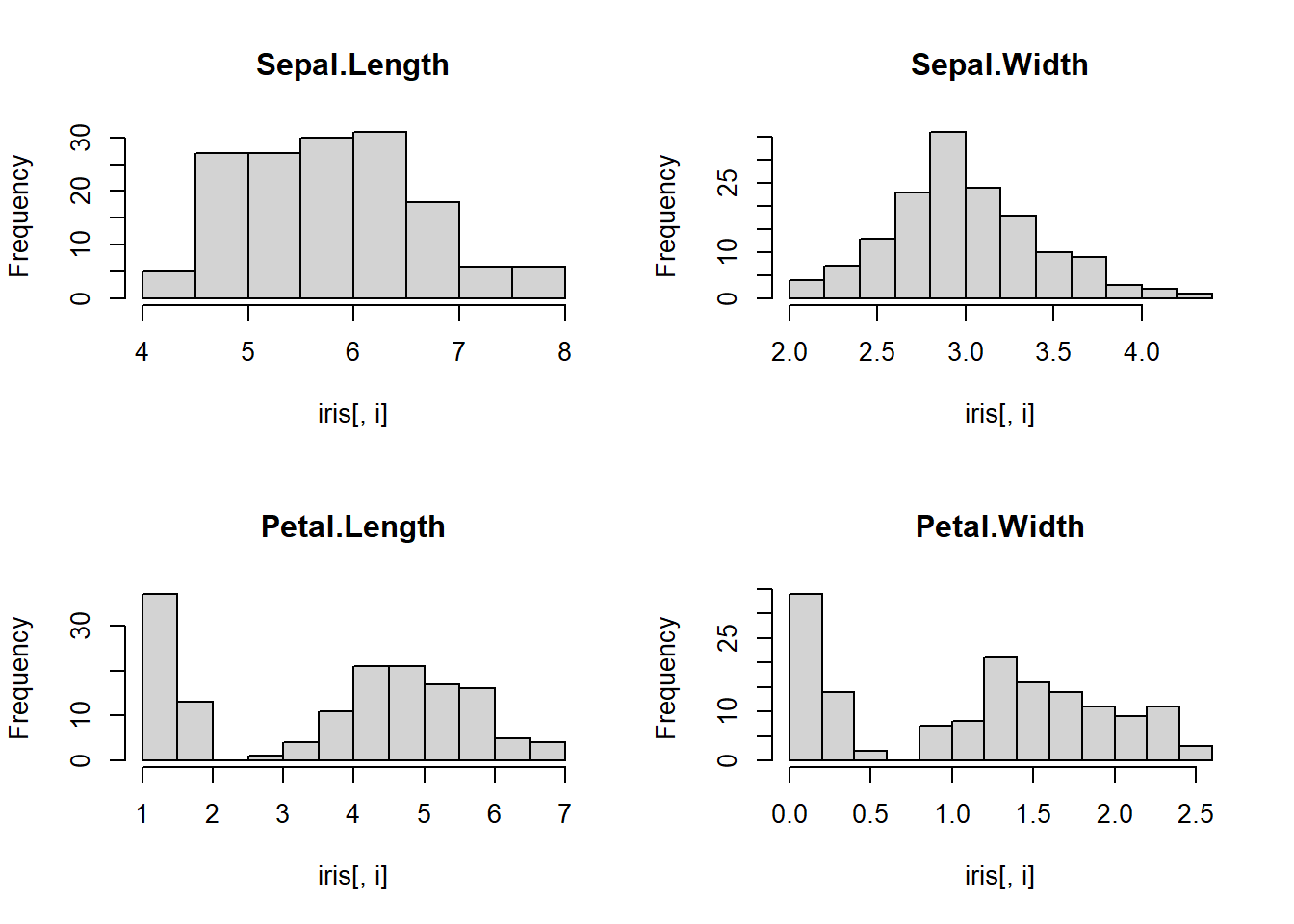## Optimal Legend Location

The function checkForLegLoc() allows to check which corner of a given graph is less crowded for placing a legend there. Basic legends can be added directly, or one can simply recuperate the location information.

``````dat1 <- matrix(c(1:5,1,1:5,5), ncol=2)
grp <- c("abc","efghijk")
(legLoc <- checkForLegLoc(dat1, grp))
#> \$showL
#>  TRUE
#>
#> \$loc
#>  "bottomright"
#>
#> \$nConflicts
#>     topleft    topright bottomright  bottomleft
#>           1           1           0           1

# now with more graphical parameters (using just the best location information)
plot(dat1, cex=2.5, col=rep(2:3,3),pch=rep(2:3,3))
legLoc <- checkForLegLoc(dat1, grp, showLegend=FALSE)
legend(legLoc\$loc, legend=grp, text.col=2:3, pch=rep(2:3), cex=0.8)``````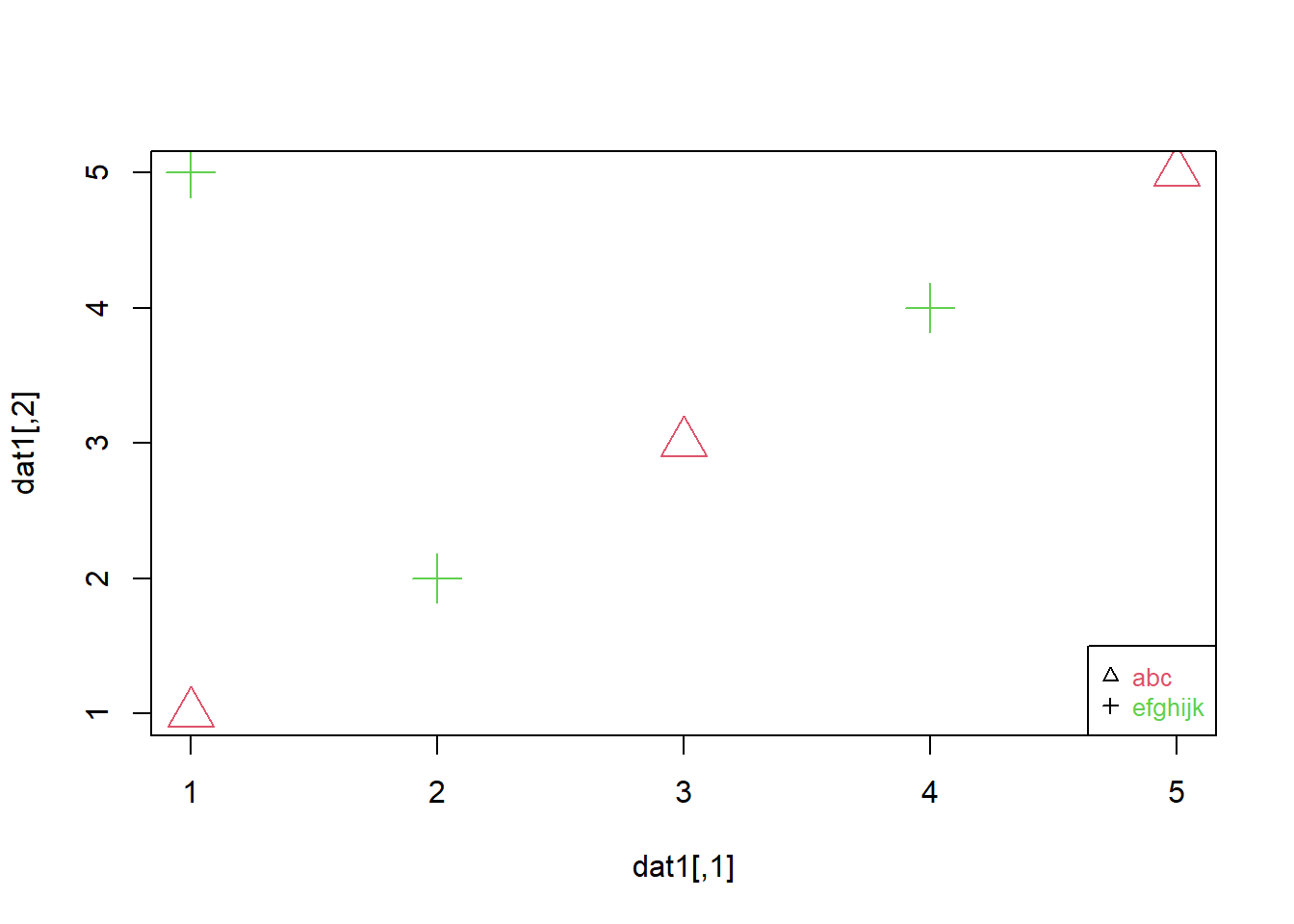## One More Histogram Function …

Histograms are a very versatile tool for rapidly gaining insights in the distribution of data. This package presents a histogram function allowing to conveniently work with log2-data and (if desired) display numbers calculated back to linear values on the x-axis.

Default settings aim to give rather a quick overview, for “high resolution” representations one could set a high number of breaks or one might also consider other/alternative graphical representations. Some of the alternatives are shown later in this vignette.

``````set.seed(2016); dat1 <- round(c(rnorm(200,6,0.5), rlnorm(300,2,0.5), rnorm(100,17)),2)
dat1 <- dat1[which(dat1 <50 & dat1 > 0.2)]
histW(dat1, br="FD", isLog=FALSE)``````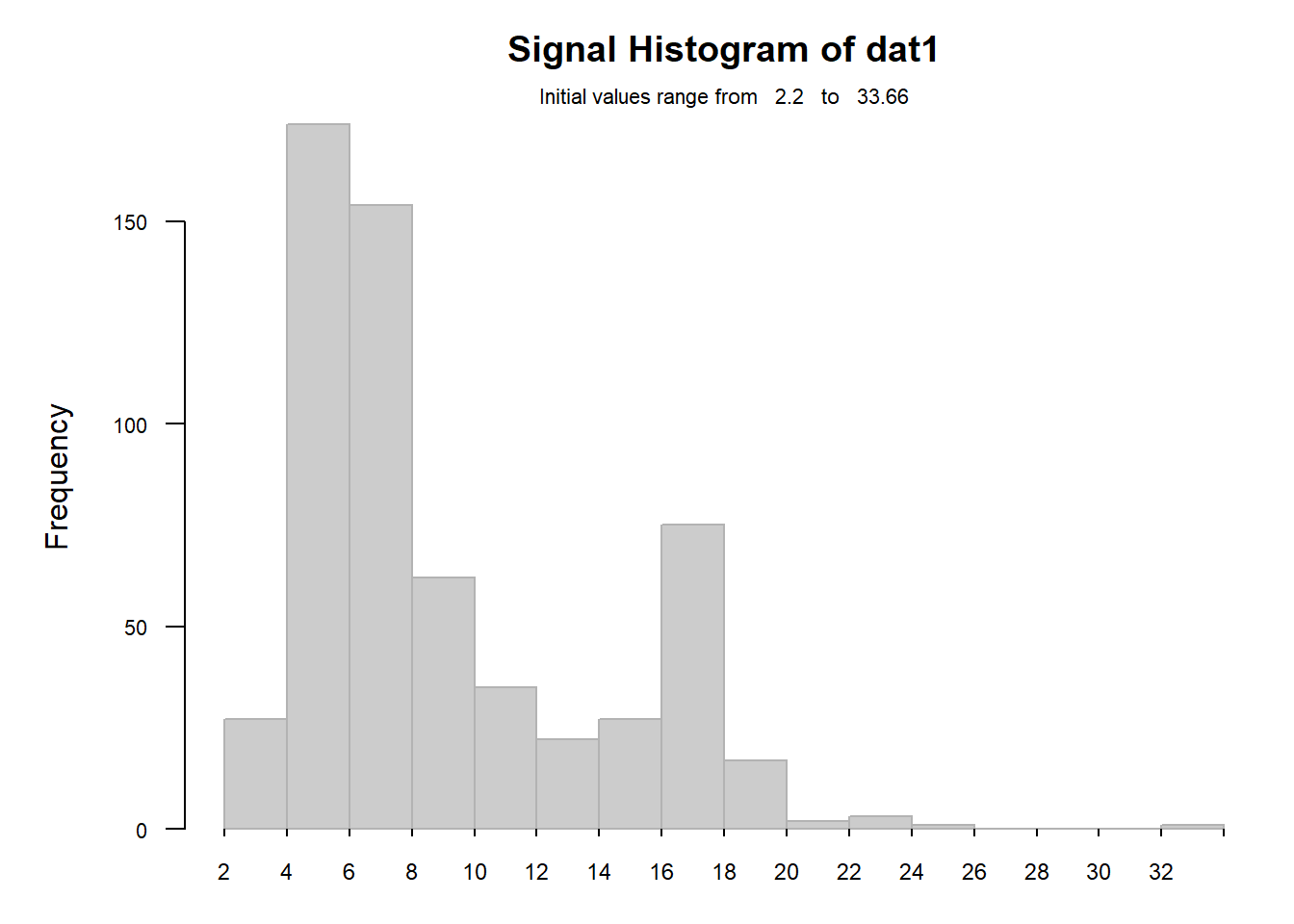One interesting feature is the fact that this fucntions can handle log-data (and display x-axis classes as linear) :

``````## view as log, but x-scale in linear
histW(log2(dat1), br="FD", isLog=TRUE, silent=TRUE)``````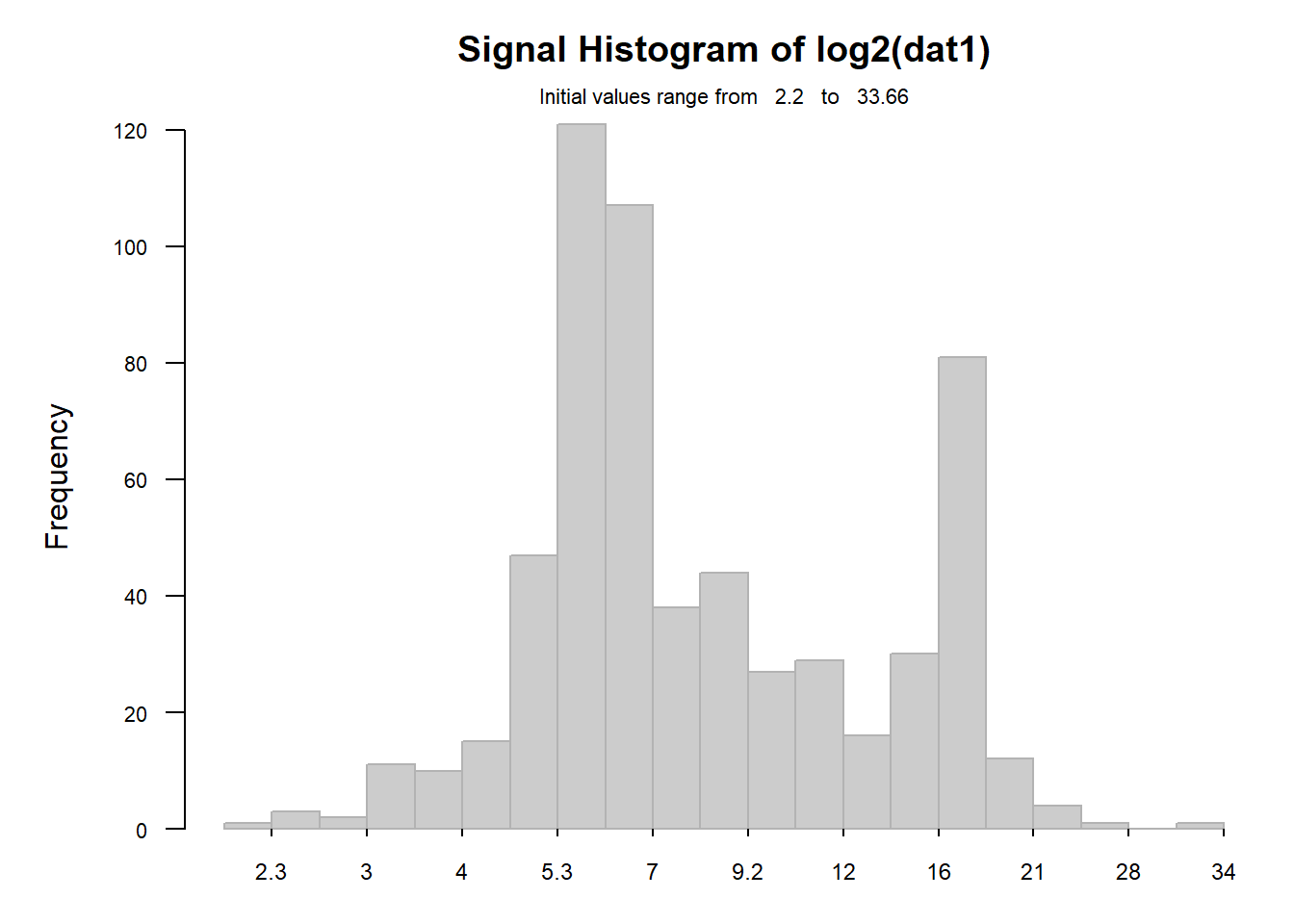Now we can combine this with the previous segmentation to accomodate 4 histograms :

``````## quick overview of distributions
layout(partitionPlot(4))
for(i in 1:4) histW(iris[,i], isLog=FALSE, tit=colnames(iris)[i])``````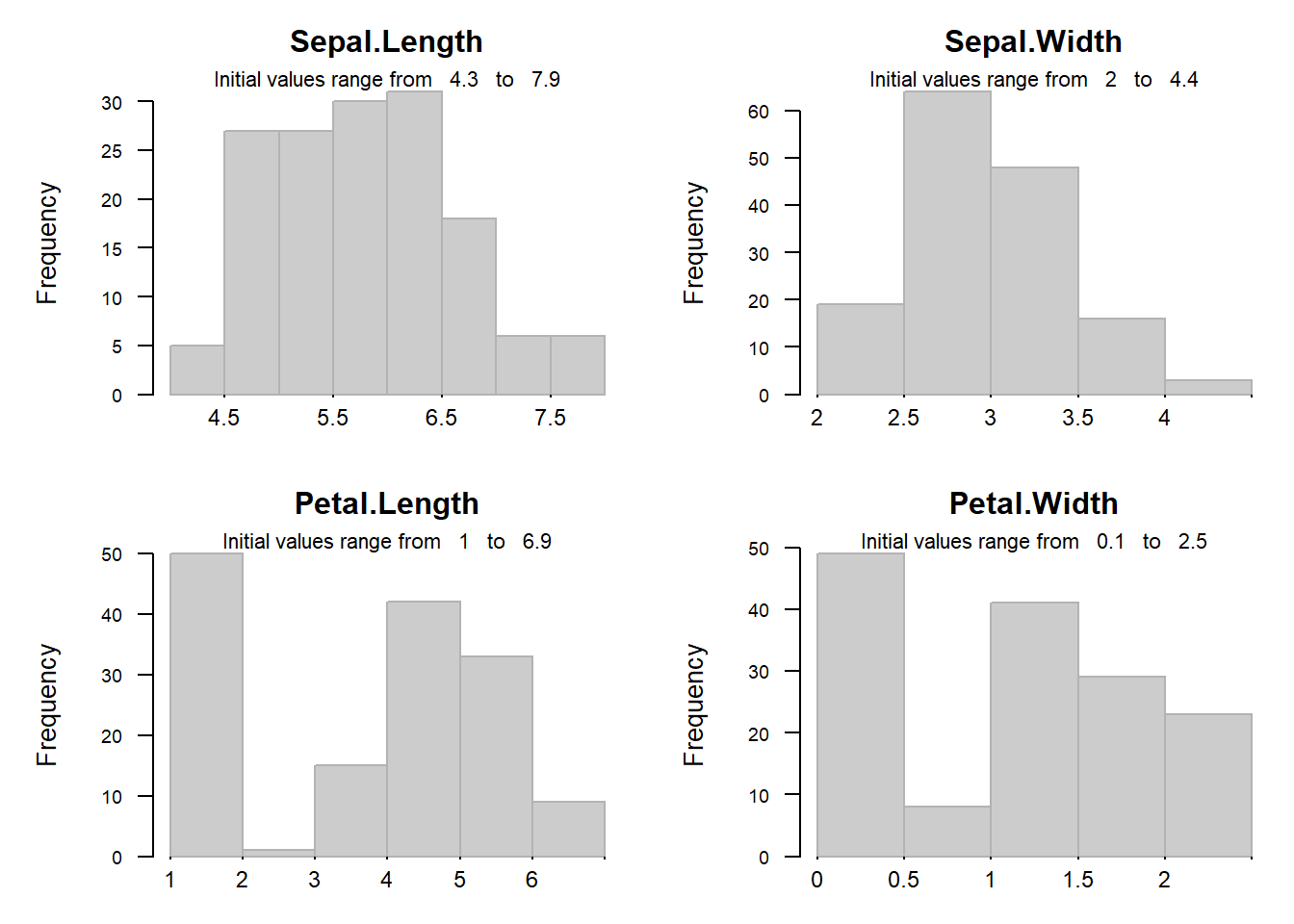### Small Histogram(s) as Legend

With some plots it may be useful to add small histograms for the x- and/or y-data.

``````layout(1)
plot(iris[,1:2], col=rgb(0.4,0.4,0.4,0.3), pch=16, main="Iris Data")
legendHist(iris[,1], loc="br", legTit=colnames(iris), cex=0.5)
legendHist(iris[,2], loc="tl", legTit=colnames(iris), cex=0.5)``````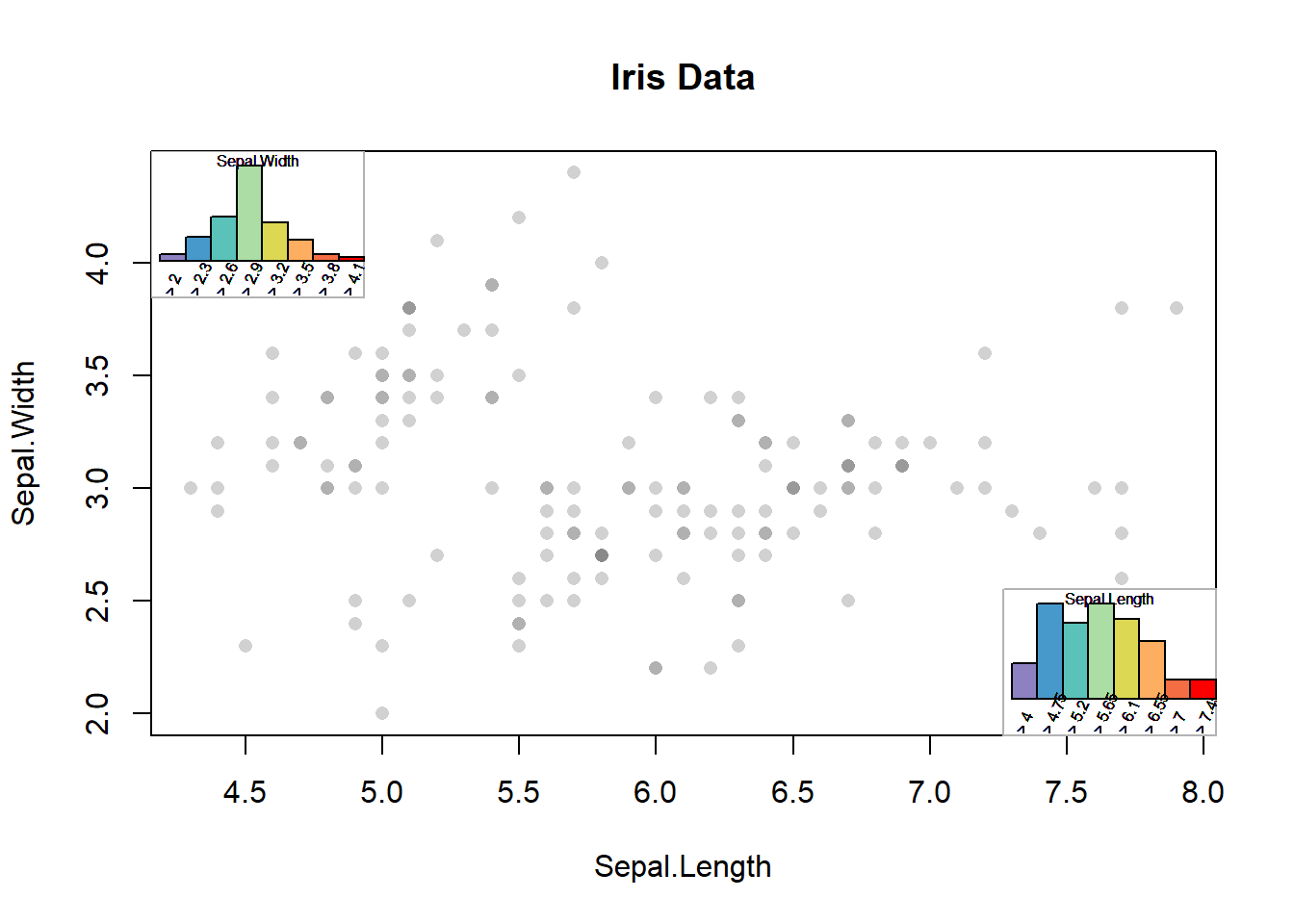## Violin Plots

Violin plots or vioplots are basically an adaptation of plotting the Kernel density estimation allowing to compare multiple data-sets. Please note, that although smoothed distributions please the human eye, some data-sets do not have such a continuous character.

Compared to the ‘original’ vioplots in R from package vioplot, the function provided here offers more flexibility for data-formats accepted (including data.frames and lists), coloring and display of n. In the case of the Iris-data, there are no NAs and thus n is constant, thus the number of values (n) will be displayed only once. However, when working with data-sets containing NAs, or simply when working with lists the number of values per data-set/violin n may vary.

``vioplotW(iris[,-5], tit="Iris-Data Violin Plot")``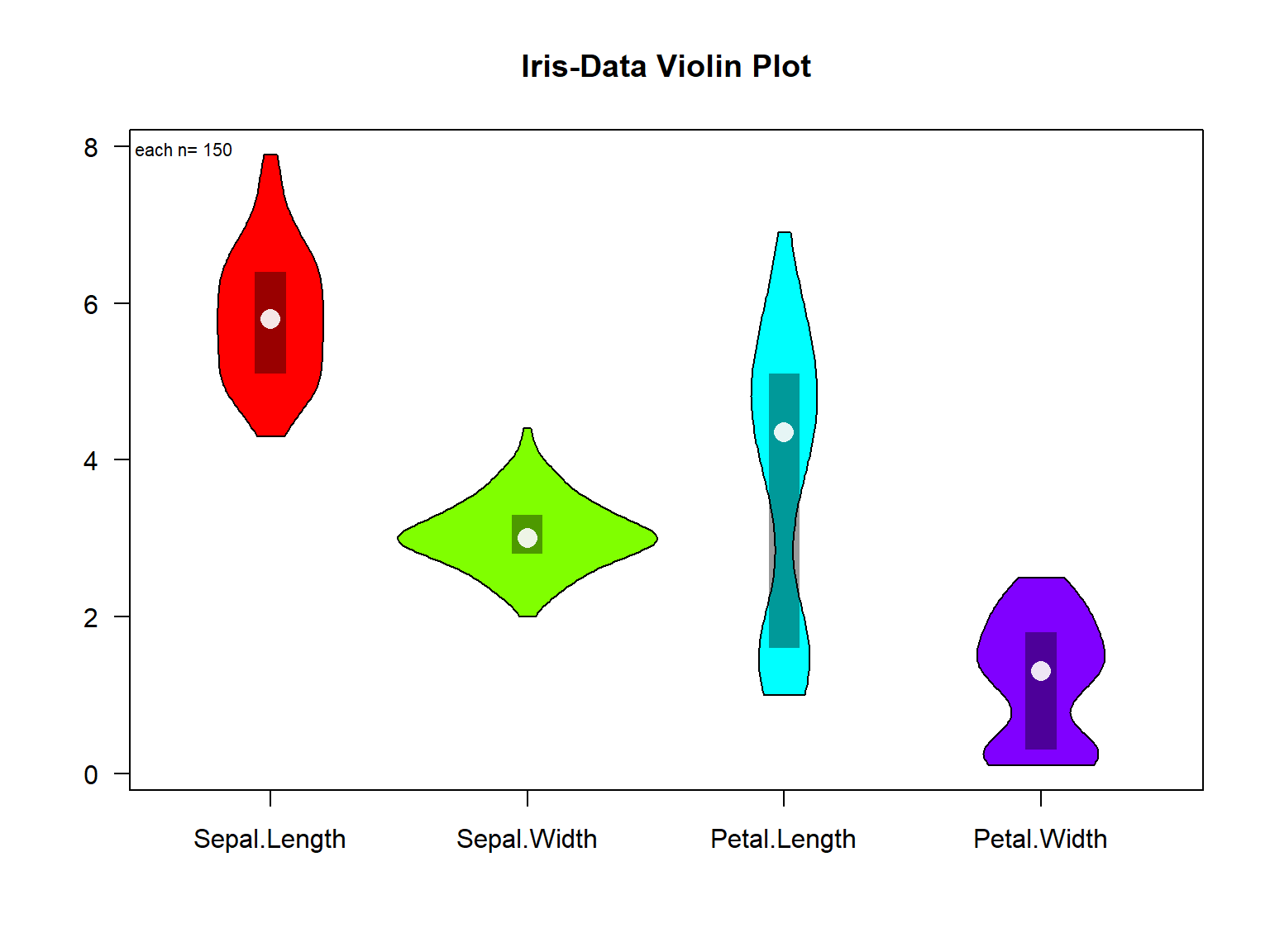The smoothing of the curves uses default parameters from the function sm.density from the package sm. In some cases the Kernel smoothing may appear to strong, this behaviour can be modified using the argument hh (which is passed on as argument h to the function sm.density).

``````## less smoothing
vioplotW(iris[,-5], tit="Iris-Data Violin Plot ('nervous')", hh=0.15)``````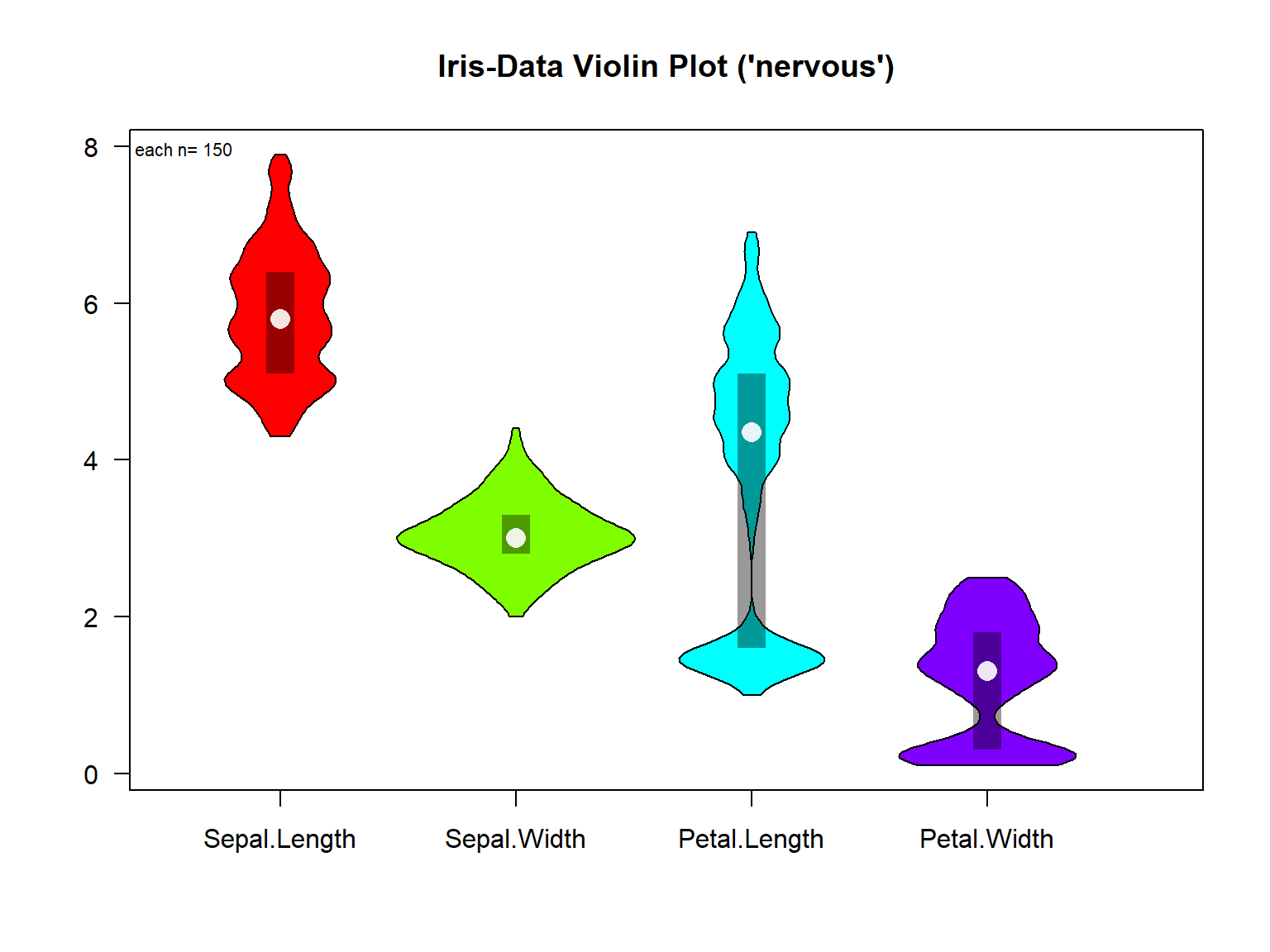## Plotting Sorted Values (‘Summed Frequency’)

This plot offers an alternative to histograms and density-plots. While histograms and density-plots are very intuitive, their interpretation may pose some difficulties due to the smoothing effect of Kernel-functions or the non-trivial choice of optimal width of bars (histogram) may influence interpretation.

As alternative, a plot is presented which basically reads like a summed frequency plot and has the main advantage, that all points of data may be easily displayed. Thus the resultant plot does not suffer from deformation due to binning or smoothing and offers maximal ‘resolution’.

``cumFrqPlot(iris[,1:4])``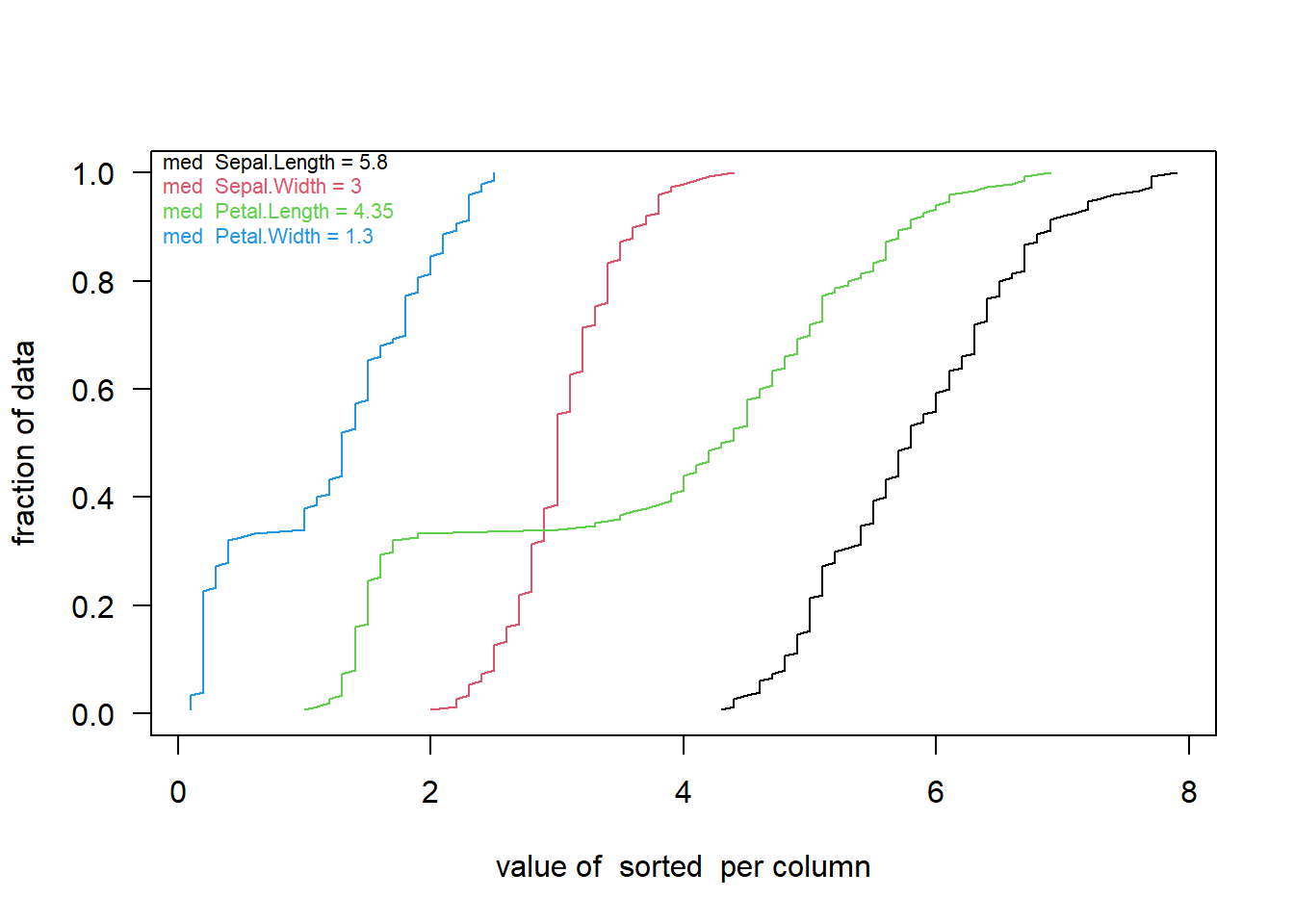For example, the Iris-data are rounded. As a result in the plot above the line is not progressing smooth but with more marked character of steps of stairs. To get the same conclusion one would need to increase the number of bars in a histogram very much which would makes it in our experience more difficult to evaluate the same time the global distribution character.

At this plot you may note that the curves patal.width and petal.length look differently. On the previous vioplots you may have noticed the bimodal character of the values, again this plot may be helpful to identify distributions which are very difficult to see well using boxplots.

## Color-Code Numeric Content of Matrix (Heatmap)

To get a quick overview of the distribution of data and, in particular, of local phenomena it is useful to express numeric values as colored boxes. The function image() from the graphic-package provides basic help.

Generally this type of display is called heatmap, however, most functions in R combine this directly with organizing by hierarchical clustering (heatmap() (package stats) or heatmap.2() from package gplots).

Simple plotting without reorganizing rows and columns can be done using the function imageW() from this package, offering convenient options for displaying row- and column-names. Using the argument transp you can decide if the data should be shown as is or rotated by 90 degrees (as in example below). Furthermore, the output can be produced using stadard graphics or using the trellis/lattice framework. The latter includes also an automatic legend for the color-codes. Below, the first 40 lines of the Iris-dataset are used :

``````par(mar=c(4, 5.5, 4, 1))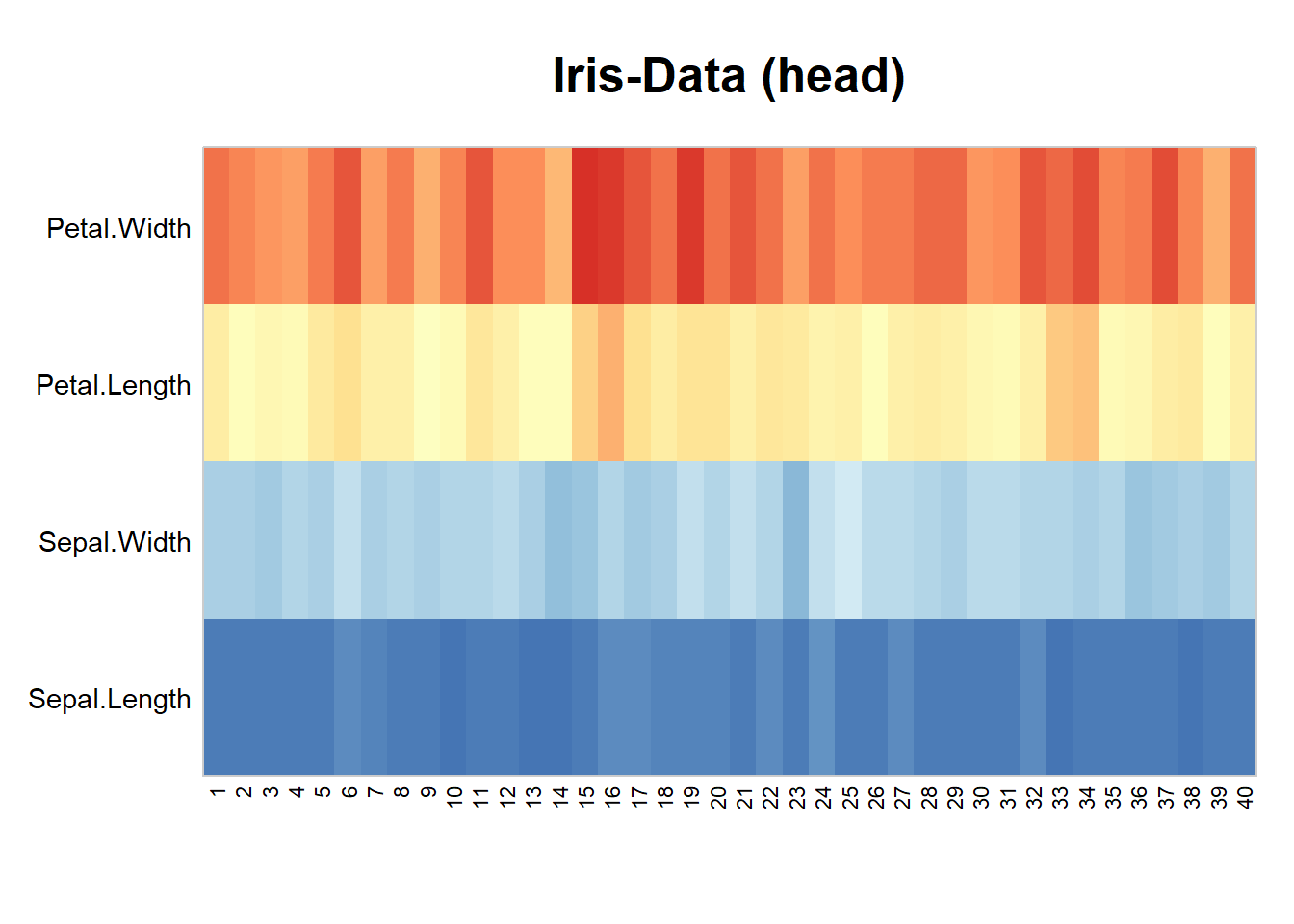Here again the Iris-data plotted using the lattice/trellis framework :

``````imageW(as.matrix(iris[1:20,1:4]), latticeVersion=TRUE, transp=FALSE, col=c("blue","red"),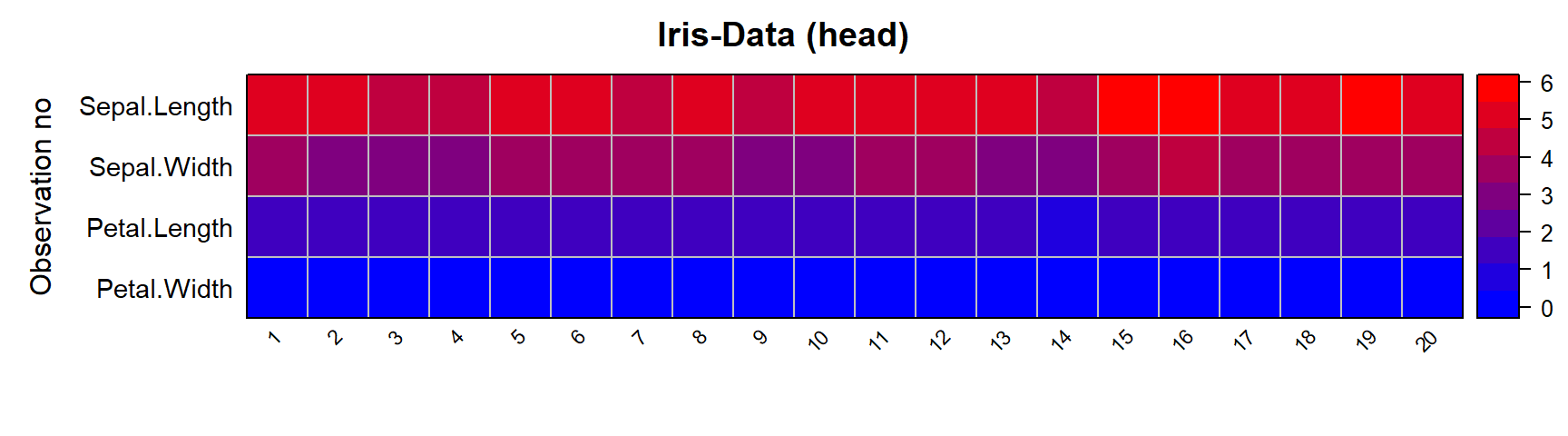Note, by default this version forces the aspect argument of levelplot() to square shapes. In some cases it may be desirable to pass the color-gradient through the value of 0 at a predefined color (use the center element of tha argument col). One can also display the (rounded) values and choose a custom color for NA-values.

``````ma1 <- matrix(-7:16,nc=4,dimnames=list(letters[1:6],LETTERS[1:4]))
ma1[1,2:3] <- 0
ma1[3,3] <- ma1[3:4,4] <- NA

imageW(ma1, latticeVersion=TRUE, col=c("blue","grey","red"), NAcol="grey92",
rotXlab=0, cexDispl=0.8, tit="Balanced color gradient")   ``````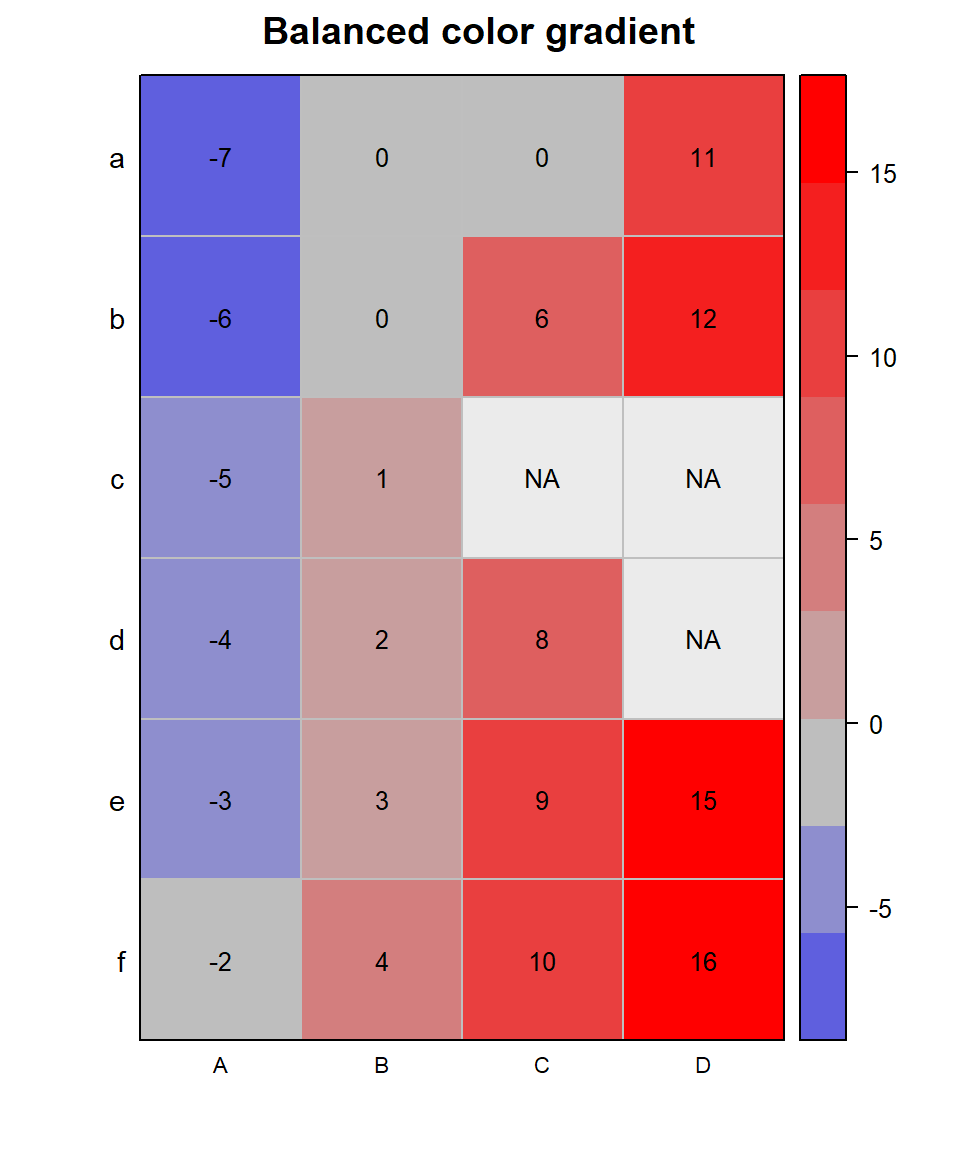By changing the number of desired color-steps we can get the value of 0 better centered to grey color. Below, the value of +1 is shown in grey as -1 in contrast to the example above.

``````imageW(ma1, latticeVersion=TRUE, col=c("blue","grey","red"), NAcol="grey92",
rotXlab=0, nColor=8, cexDispl=0.8, tit="Balanced color gradient")   ``````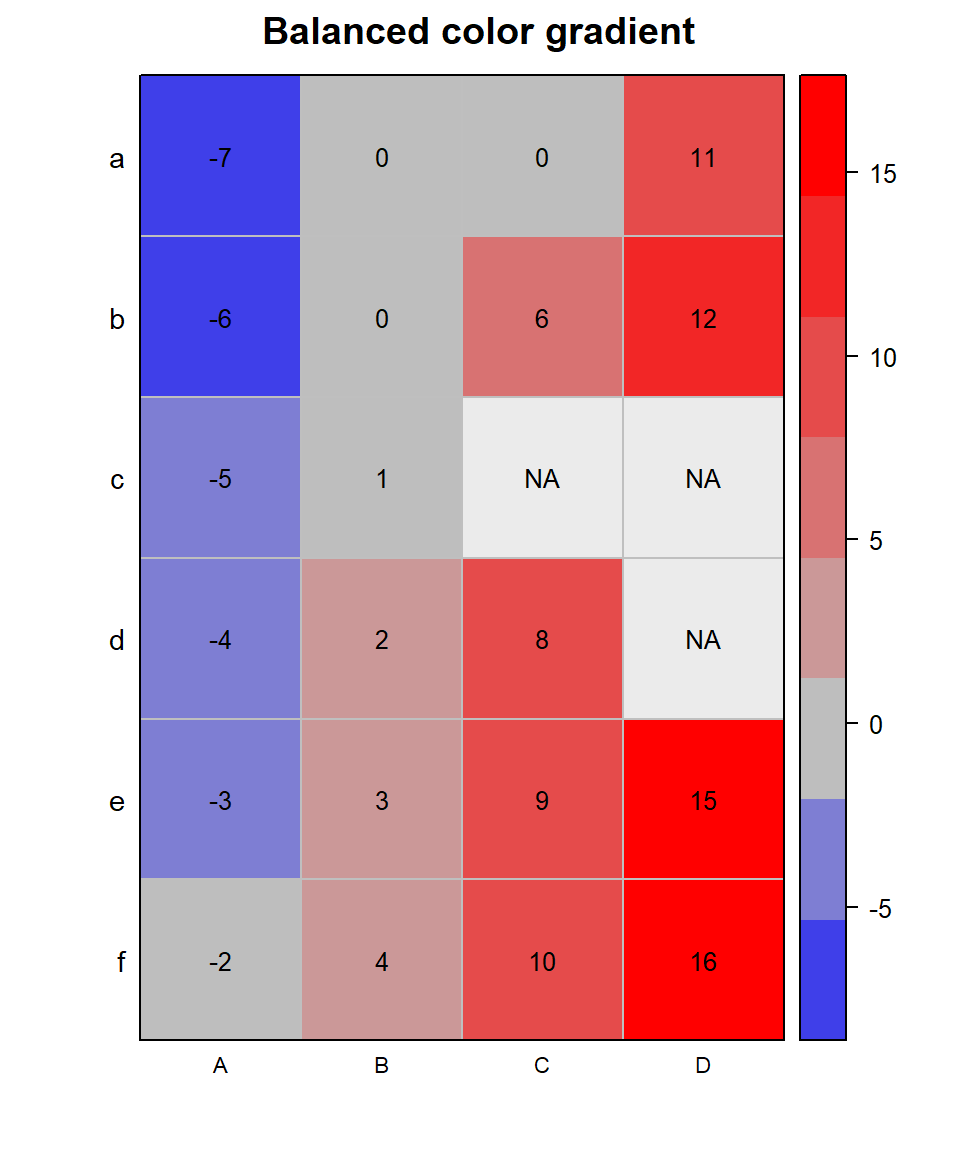## Examine Counts based on Variable Threshold Levels (for ROC curves)

The next plot is dedicated to visualize counting results with moving thresholds. While a given threshold criteria moves up or down the resulting number of values passing may not necessarily follow in a linear way. This function lets you follow multiple types of samples (eg type of leave in the Iris-data) in a single plot. In particular when constructing ROC curves it may also be helpful to visualize the (absolute) counting data used underneath before determining TP and FP ratios. Typically used in context of benchmark-tests in proteomics.

``````thr <- seq(min(iris[,1:4]), max(iris[,1:4])+0.1,length.out=100)
irisC <- sapply(thr,function(x) colSums(iris[,1:4] < x))
irisC <- cbind(thr,t(irisC))

#>            thr Sepal.Length Sepal.Width Petal.Length Petal.Width
#> [1,] 0.1000000            0           0            0           0
#> [2,] 0.1797980            0           0            0           5
#> [3,] 0.2595960            0           0            0          34
#> [4,] 0.3393939            0           0            0          41
#> [5,] 0.4191919            0           0            0          48
#> [6,] 0.4989899            0           0            0          48
staggerdCountsPlot(irisC[,], countsCol=colnames(iris)[1:4], tit="Iris-Data")``````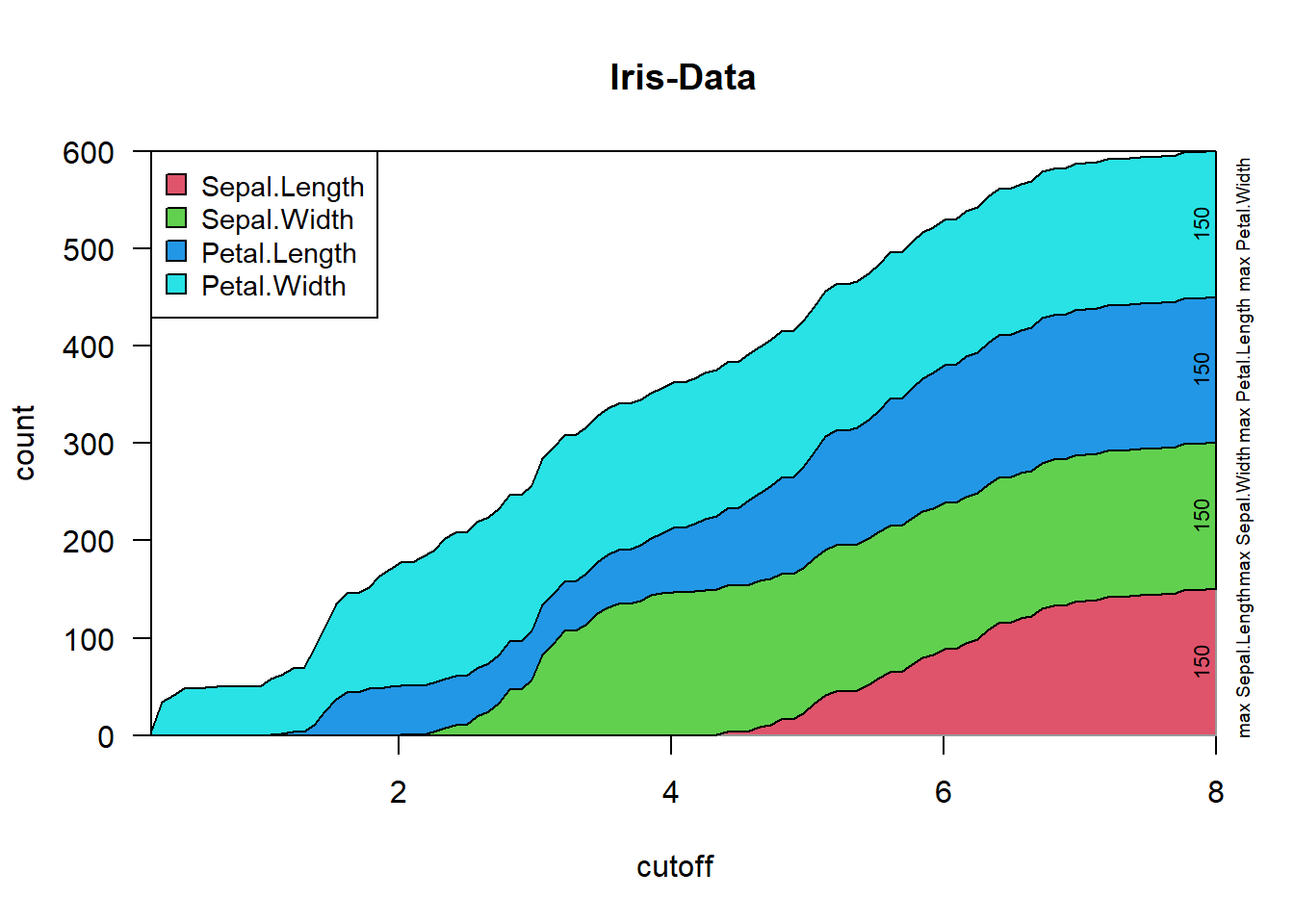``staggerdCountsPlot(irisC[,], varCountNa="Sepal", tit="Iris-Data")``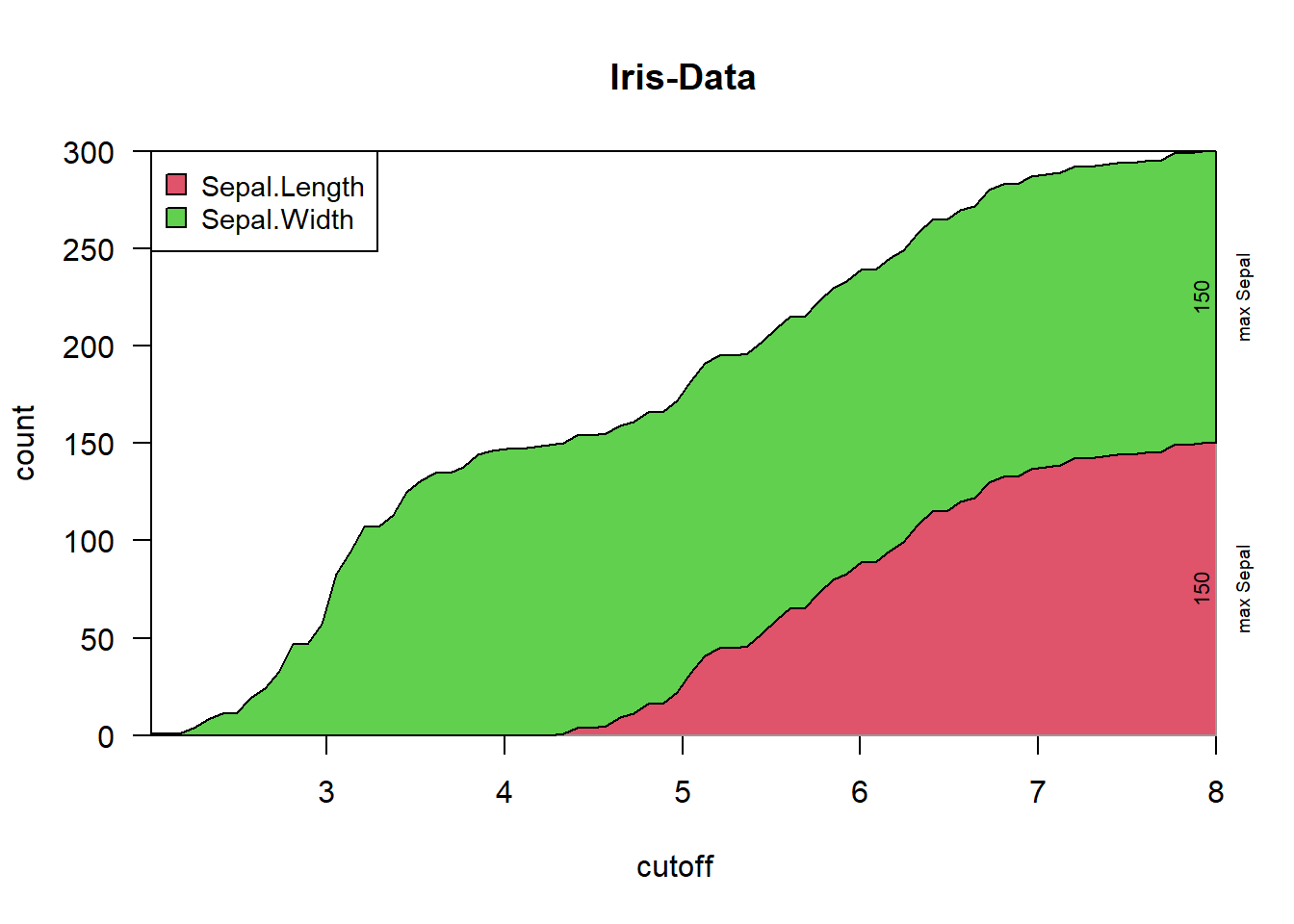``staggerdCountsPlot(irisC[,], varCountNa="Sepal", tit="Iris-Data (log-scale)", logScale=TRUE)``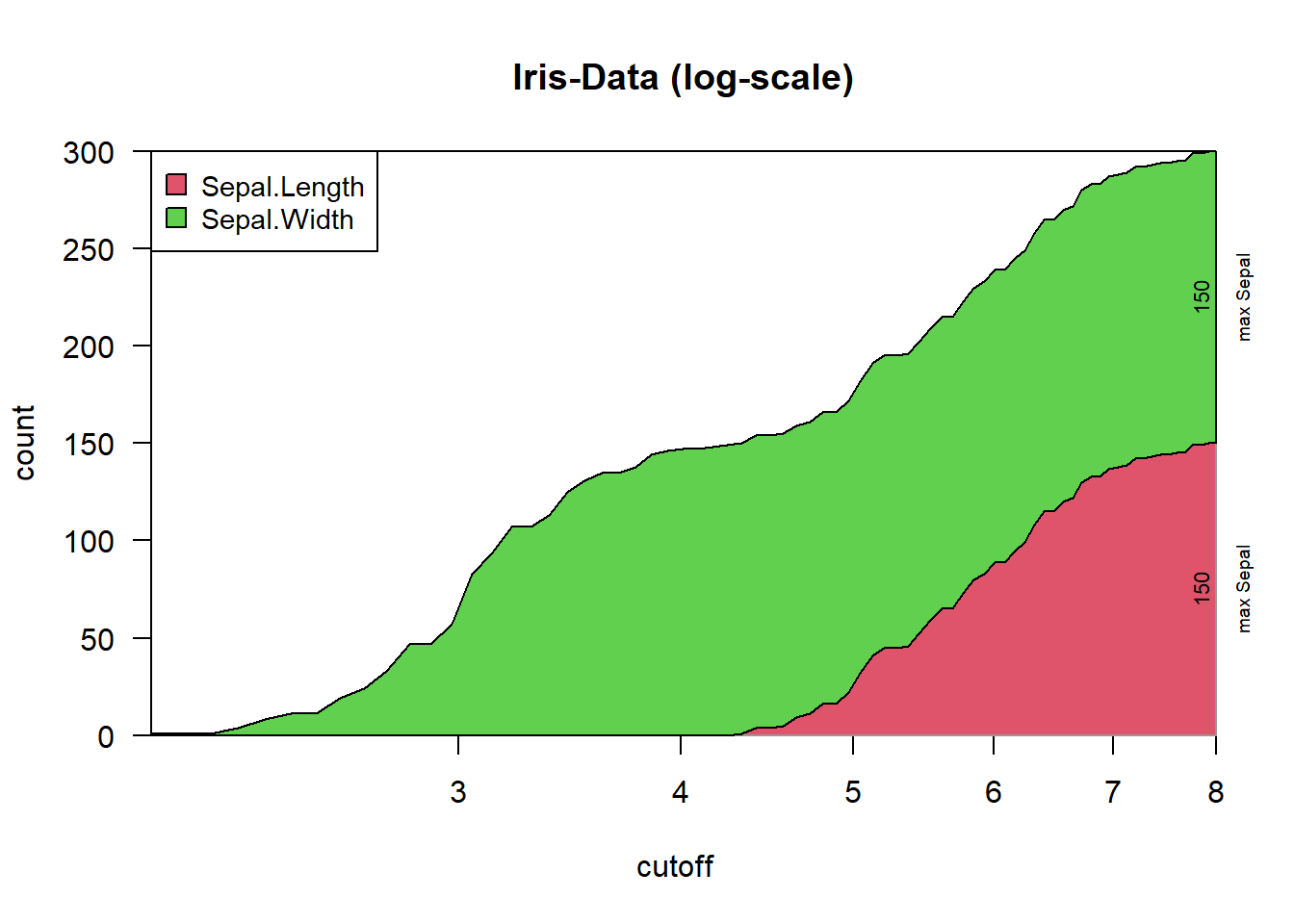## Compare Two Groups with Sub-Organisation Each

In real-world testing data have often some nested structure. For example repeated measures from a set of patients which can be organized as diseased and non-diseased. This plot allows to plot all values obtained from the each patient together, then organized by disease-groups.

For this example suppose the Iris-data were organized as 10 sets of 5 measures each (of course, in this case it is a pure hypothesis). Then, we can plot while highlighting the two factors (ie species and set of measurement). Basically we need to furnish with the main data two additional factors for the groupings. Note, that the 1st factor should contain the smaller sub-groups to visually inspect if there are any batch effects. This plot is not well adopted to big data (it will get too crowded).

``````dat <- iris[which(iris\$Species %in% c("setosa","versicolor")),]
plotBy2Groups(dat\$Sepal.Length, gl(2,50,labels=c("setosa","versicolor")),
gl(20,5), yLab="Sepal.Length")``````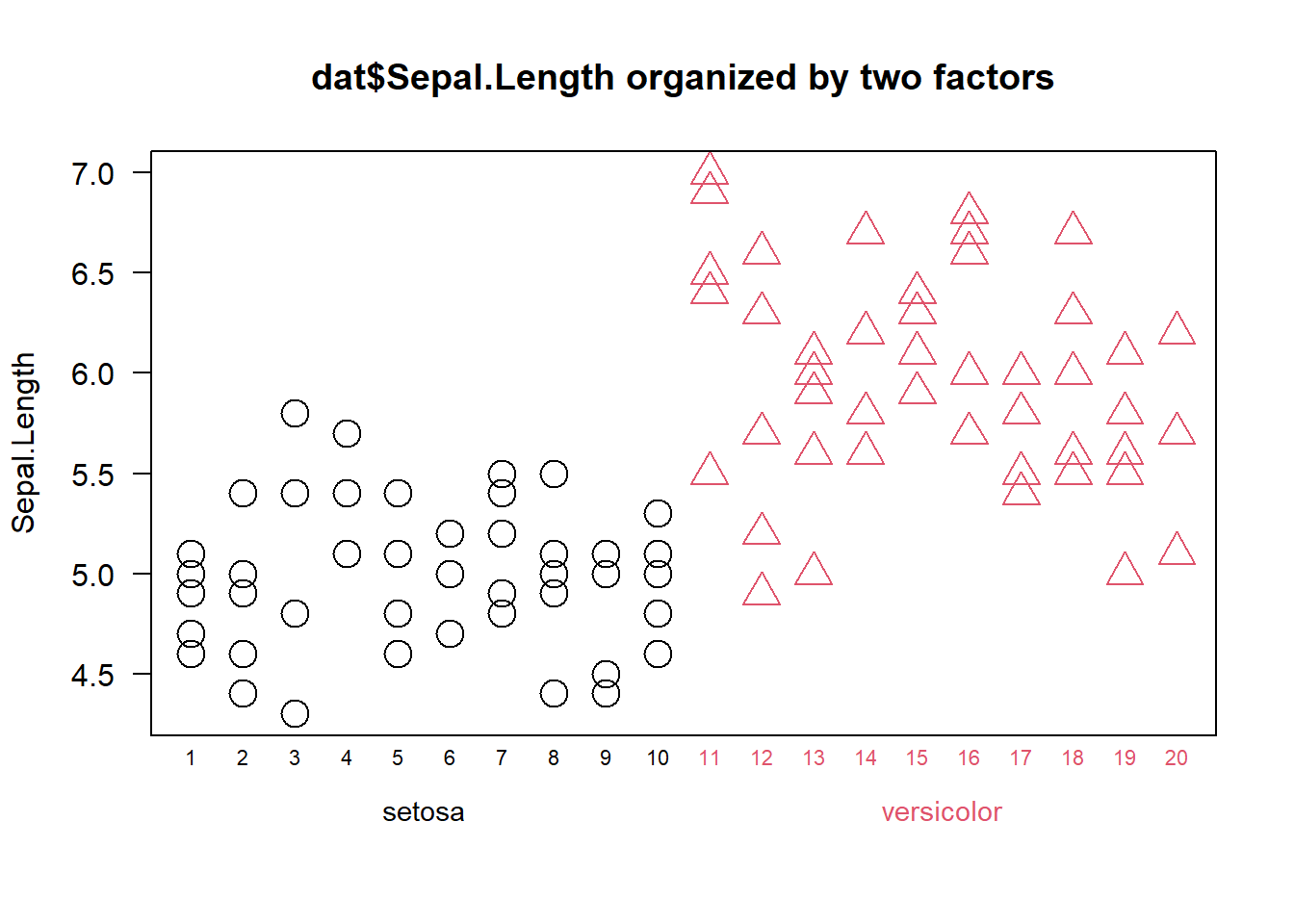## Plotting Linear Regression and Confidence Intervals

The function plotLinReg() provides help to display a series of bivariate points given in ‘dat’ (multiple data formats possible), to model a linear regression and plot the results.

``plotLinReg(iris\$Sepal.Length, iris\$Petal.Width, tit="Iris-Data")``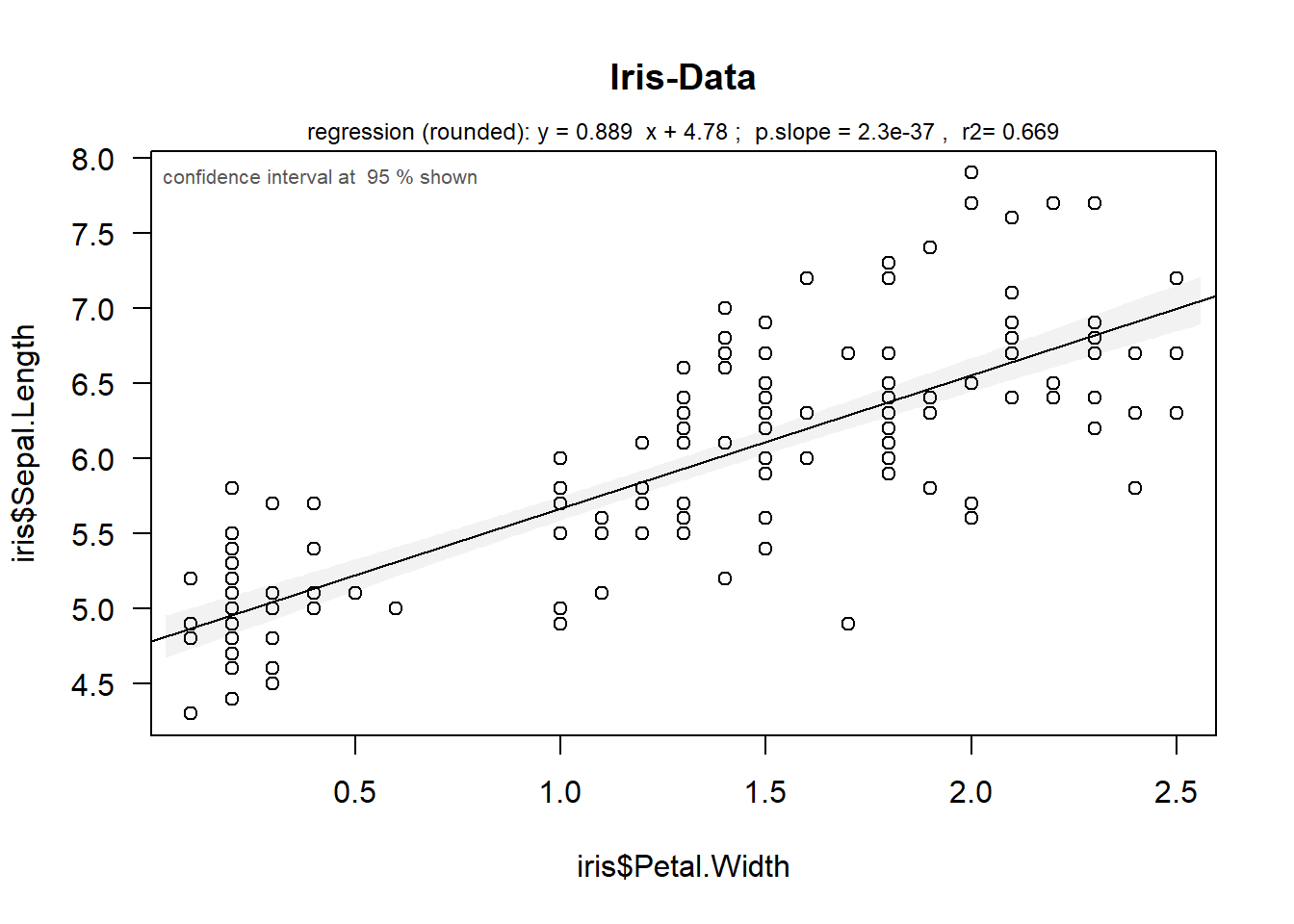## Principal Components Analysis (PCA)

Principal components analysis, PCA, is a very powerful method to investigate similarity and correlation in larger sets of data.
Please note that several implementations exist in R (eg prcomp() in the base package stats or the package FactoMineR). We’ll start by looking at the plot produced with the basic function and FactoMineR, too.

Let’s look at the similarity of the 3 Iris-species from the Iris data-set.

``````## the basic way
iris.prc <- prcomp(iris[,1:4], scale.=TRUE)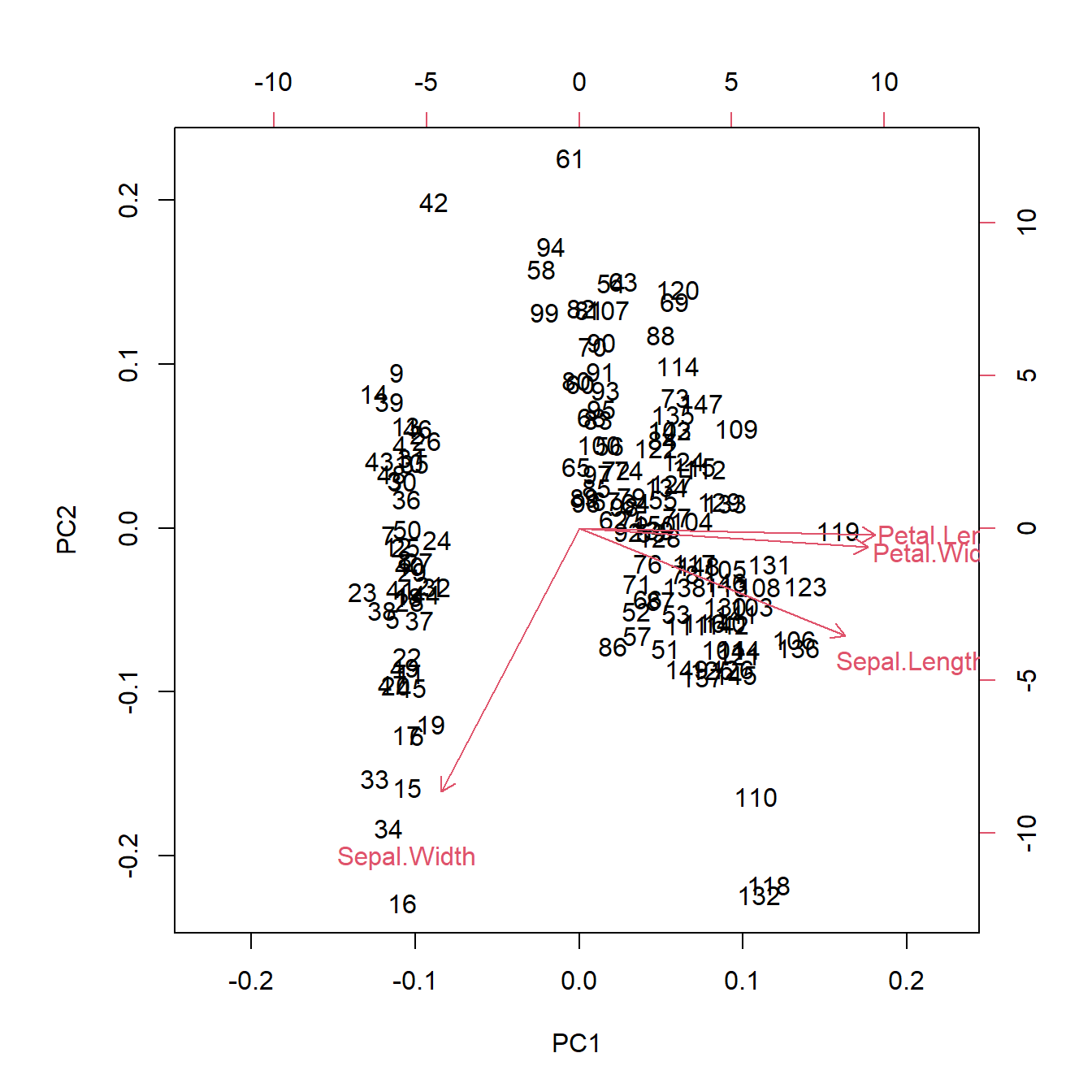``````## via FactoMineR
chPa <- c(requireNamespace("FactoMineR", quietly=TRUE), requireNamespace("dplyr", quietly=TRUE),
requireNamespace("factoextra", quietly=TRUE))
if(all(chPa)) {
library(FactoMineR); library(dplyr); library(factoextra)
iris.Fac <- PCA(iris[,1:4],scale.unit=TRUE, graph=FALSE)
fviz_pca_ind(iris.Fac, geom.ind="point", col.ind=iris\$Species, palette=c(2,4,3),
} else message("You need to install packages 'dplyr', 'FactoMineR' and 'factoextra' for this figure")
#>
#> Attaching package: 'dplyr'
#> The following objects are masked from 'package:stats':
#>
#>     filter, lag
#> The following objects are masked from 'package:base':
#>
#>     intersect, setdiff, setequal, union``````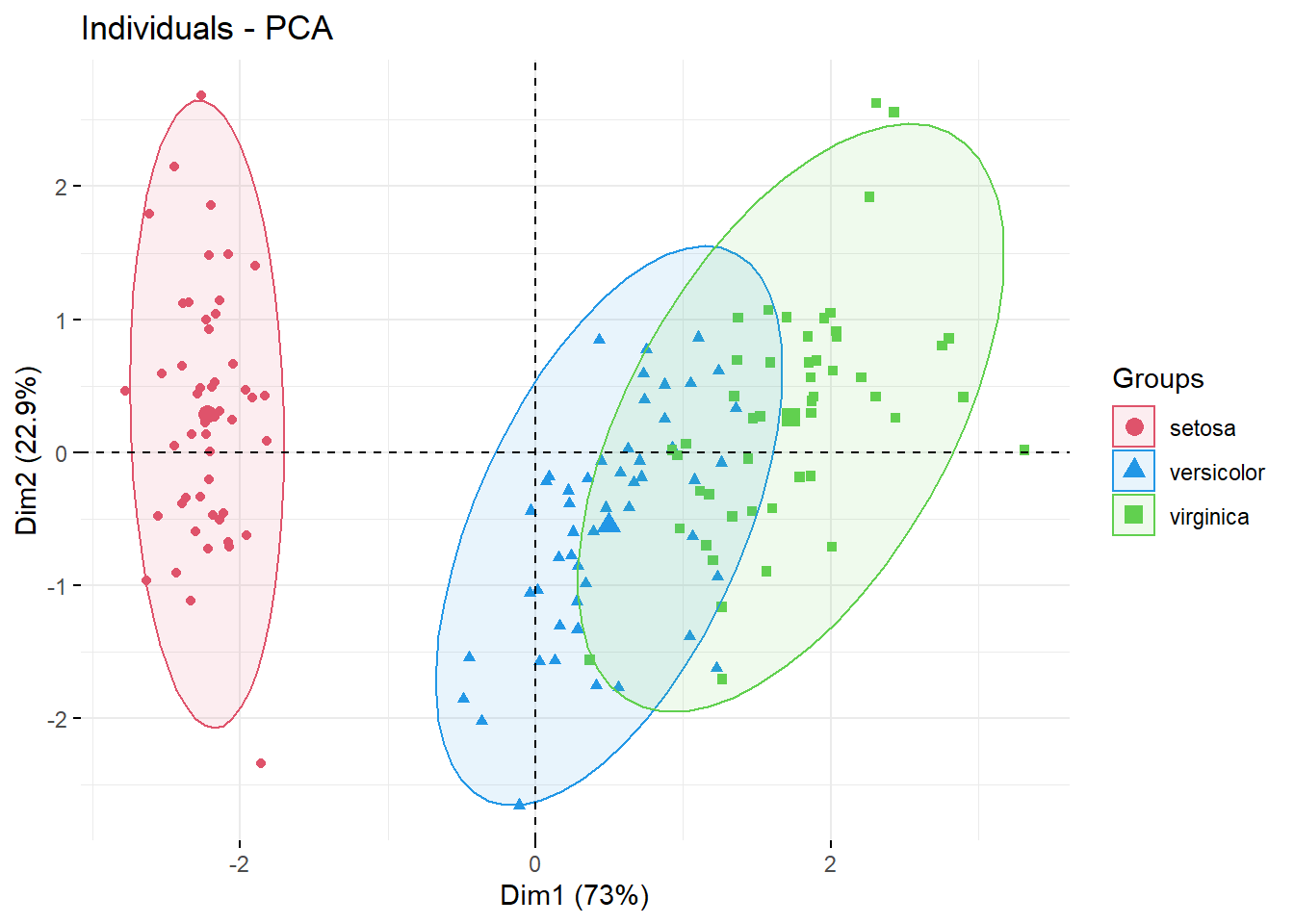However, some sets of points do not always follow elliptic shapes. Note, that FactoMineR represented the 2nd principal component upside-down compared to the very first PCA figure. To facilitate comparisons, the function plotPCAw has an argument allowing to rotate/flip any principal component axis.

With more crowded data-sets it may be useful to rather highlight the more dense regions. For this reason this package proposes to use bagplots to highlight the region with 50% of data-points (in analogy to boxplots), a simple line draws the contour of the most distant points.

``````## via wrGraph, similar to FactoMineR but with bagplots
plotPCAw(t(as.matrix(iris[,-5])), gl(3,50,labels=c("setosa","versicolor","virginica")),
tit="Iris Data", rowTyName="types of leaves", suplFig=FALSE, cexTxt=1.3, rotatePC=2)``````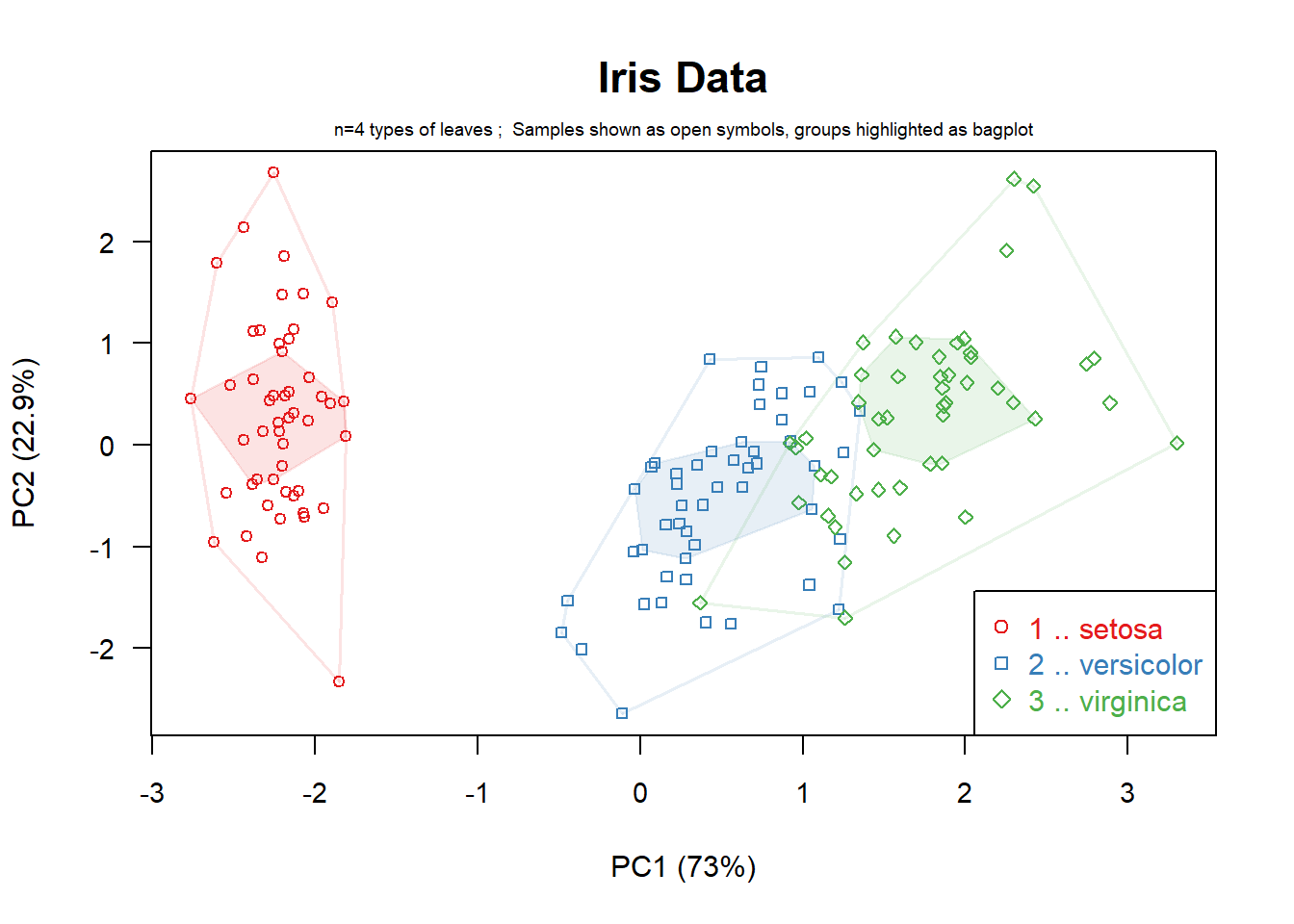Thus, you can see in this case, there is some intersection between versicolor and virginica species, but the center regions stay apart. Of course, similar to boxplots, this representation is nor adopted/recommended for multi-modal distributions within one group of points. One might get some indications about this by starting the data-analysis by inspecting histograms or vioplots for each set/column of data.

You can also add the 3rd principal component and the Scree-plot :

``````## including 3rd component and Screeplot
plotPCAw(t(as.matrix(iris[,-5])), gl(3,50,labels=c("setosa","versicolor","virginica")),
tit="Iris Data PCA", rowTyName="types of leaves", cexTxt=2)``````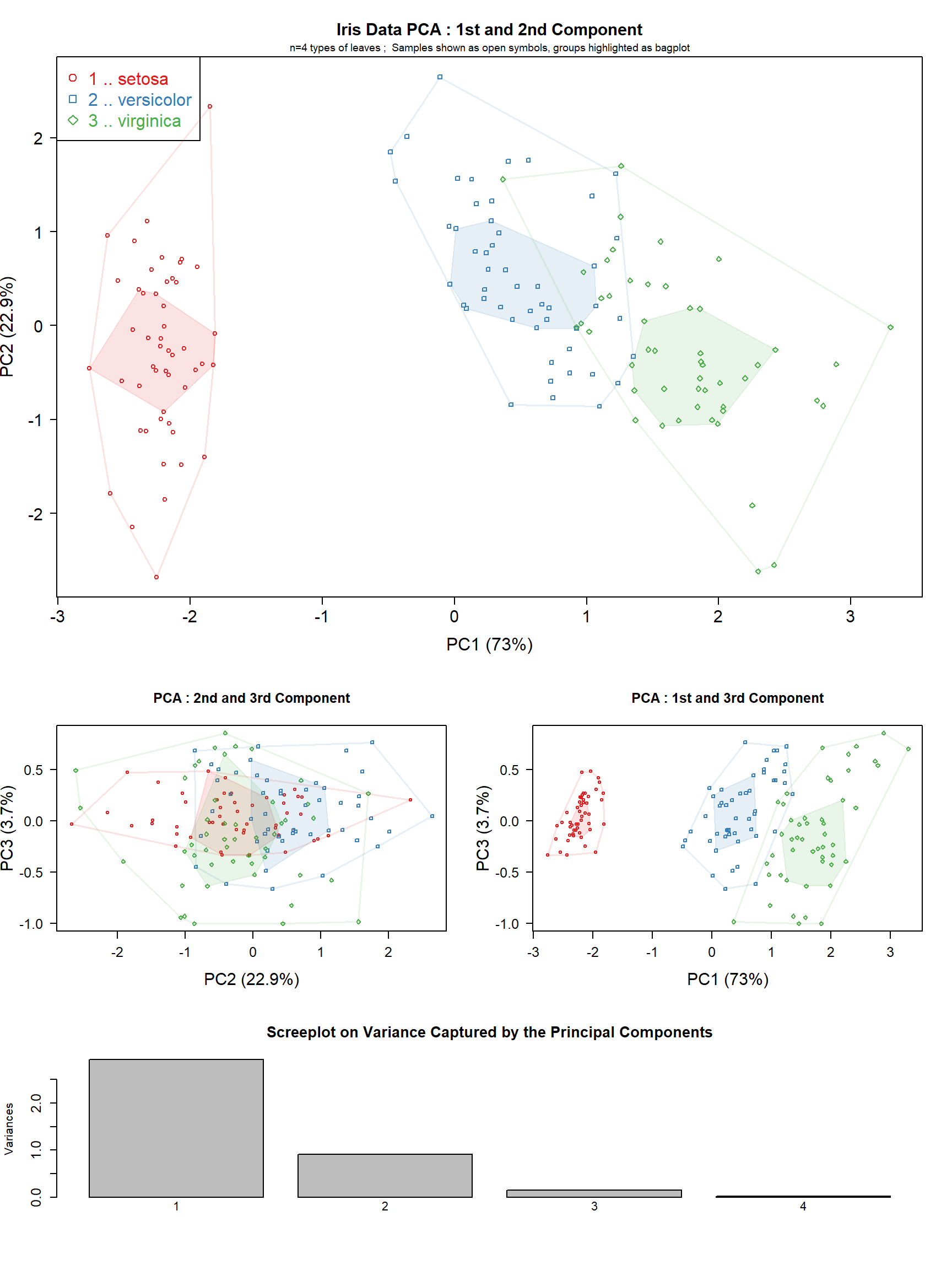### Label all points in PCA

In some cases it may be useful to identify all individual points on a plot.

``````## creat copy of data and add rownames
irisD <- as.matrix(iris[,-5])
rownames(irisD) <- paste(iris\$Species, rep(1:50,3), sep="_")
plotPCAw(t(irisD), gl(3,50,labels=c("setosa","versicolor","virginica")), tit="Iris Data PCA",
rowTyName="types of leaves", suplFig=FALSE, cexTxt=1.6, rotatePC=2, pointLabelPar=list(textCex=0.45))``````